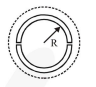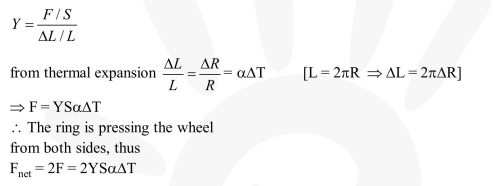# A wooden wheel of radius

Question:

A wooden wheel of radius $\mathrm{R}$ is made of two semicircular parts (see figure). The two parts are held together by a ring made of a metal strip of cross sectional area $\mathrm{S}$ and Length $\mathrm{L}$. $\mathrm{L}$ is slightly less than $2 \pi \mathrm{R}$. To fit the ring on the wheel, it is heated so that its temperature rises by $\Delta \mathrm{T}$ and it just steps over the wheel. As it cools down to surrounding temperature, it presses the semicircular parts together. If the coefficient of linear expansion of the metal is $\alpha$, and its Young's modulus is Y, the force that one part of the wheel applies on the other part is :1. $2 \mathrm{SY} \alpha \Delta \mathrm{T}$

2. $2 \pi \mathrm{SY} \alpha \Delta \mathrm{T}$

3. SY $\alpha \Delta \mathrm{T}$

4. $\pi \mathrm{SY} \alpha \Delta \mathrm{T}$

Correct Option: 1

Solution: